# Level Sets With OpenVDB. Quick Introduction. Part 1

## Intro

From the high-level of view level-set method (further just level set) can be considered as geometry representation, in addition to polygonal meshes and NURBS. This geometry representation simplifies solution of some problems in Computational Geometry, physical based modeling in Computer Graphics. Although level sets were first used for geometry tracking in 80s by Osher and Sethian, the main development of this method took place in the end of 90s and during 2000s.

OpenVDB is a new library by DreamWorks which contains data structures and tools for work with three-dimensional grids. In particular, it contains tools to work with level sets. OpenVDB is a very new library, before this library there was only one well-developed library addressing level set - Field3D. Yet it didn’t fit my needs so I wrote levelset-light library for my needs.

In order to use OpenVDB you need to build it. In case if you use Mac OS the following post might be useful for you - OpenVDB build on MacOS. Pay attention to the tools vdb_print and vdb_view in the bin folder in the OpenVDB installation directory. I will use these tools for visualization of the computation results so if you want to follow, check that they are working. In case if you are using Mac, you might have problems with vdb_view because shader language version used inside is not supported by Mac. In order to fix it, have a look inside of this patch. One more comment before we start, if you are suffering from the long compilation time of the code which uses OpenVDB, check out this discussion.

## Level sets from mathematical point of view

Level set of an implicit function is a set of points where this function has a predefined value. For instance, lets consider a function . It describes a parabola which intersects axe x in two points -1 and 1, thus the set of points where f is equal to 0 (a predefined value, can be any number) is .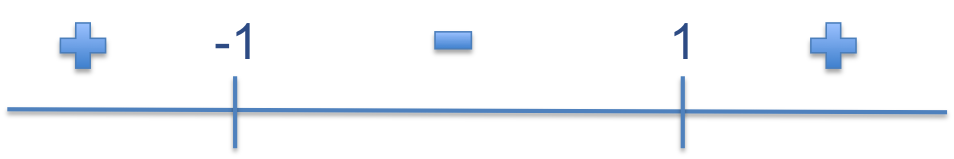In practice, a level set function is usually constructed as a signed distance function. I.e. for a given surface and an arbitrary point, we define such a function as a signed distance from the point to the surface: if the point is outside the surface the function value is positive, if it is inside - negative, and, finally, 0 if it is on the surface.

Coming back to the example above, the surface is composed of two points in 1D space: {-1, 1}, all points which belongs segment (-1, 1) are inside our surface, other - outside. We want to construct a signed distance function so, in order to start, lets get an arbitrary point 2 which is outside of the surface. The distance from this point to the surface is, obviously, 1. It means that we cannot just use . For another point 0 the distance from the point to the surface is -1. As one might guess the signed distance function for our surface is .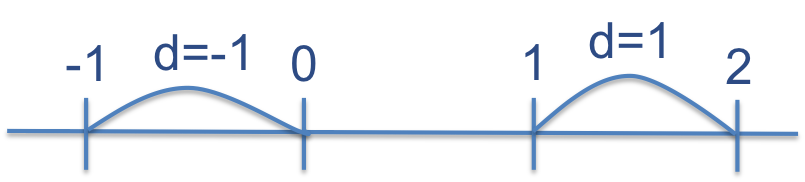1D space is not really interesting so lets move to 2D and construct a signed distance function for a circle with the center in the origin and radius R. Lets get an arbitrary point x = (x, y) outside the circle (vectors are in bold here and further). The distance from the origin to this point is basically . Thus the distance from the point to the surface is |x| - R.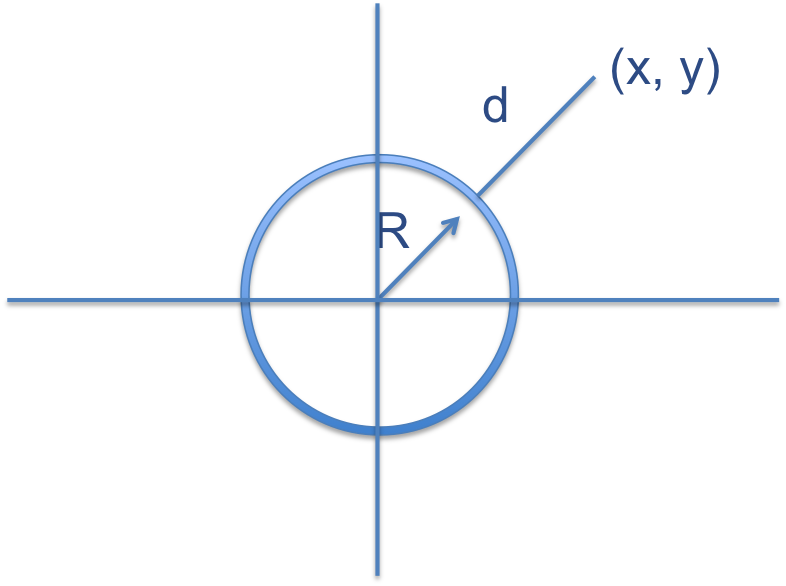In 3D space, where x=(x, y, z), the distance to the z-axis parallel cylinder surface is represented by the same function .

Following similar logic, one can write distance functions for other figures such as sphere, torus, cone, etc.

It is cool to have a new geometry representation for primitives but what if one wants to use something more complicated? E.g. surface which constructed by surfaces of two cylinders A and B. For simplicity, cylinders are z-axe oriented, so they are described by their centers and radiuses. For cylinder A, the center and the radius are denoted by , similar for cylinder B. Given an arbitrary point x outside interior of these figures, we have two distances from the cylinders: , and similar d_B. The cross-section of this two cylinder is shown below.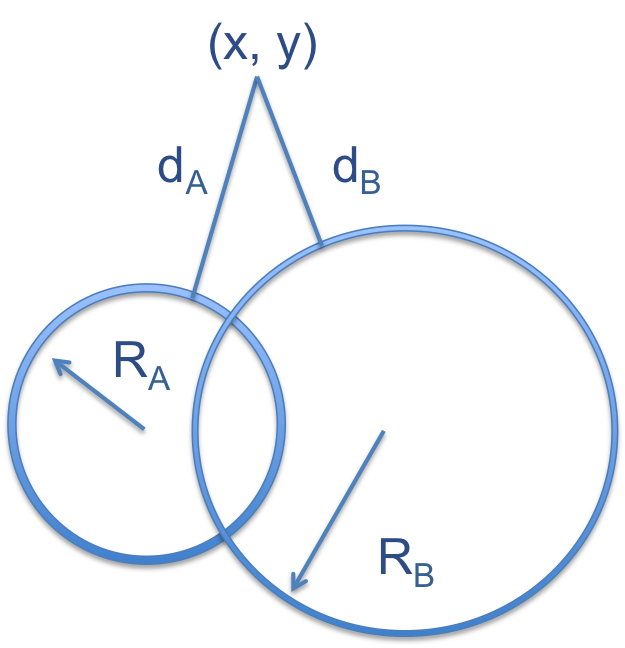Because we want to have union of this two figures, we use as a value of the distance function for x. In case if x is interior part, min will be applied to negative values and, thus, pick up the biggest in the module. Thus the part of the surface, which is inside the union of two of them, will disappear.

Other set operations on level sets are represented below:

• Union:

• Intersection:

• Complement:

• Difference (A \ B):

## From continuous level sets to discrete

Although a level set might be represented by a set of continuous level set functions and operations on them (similar to NURBS), in practice, the level set geometry is stored in a file as a grid. Every grid vertex stores the exact function value. Thus if we want to get the distance from an arbitrary point in the space to the surface, we use interpolation: find the cell of the grid to which this point belongs to and interpolate function value in this point. For instance, in 2D case for a point (x, y) we can compute a pair of indexes (i, j) which describes the cell for the point as shown on the picture below: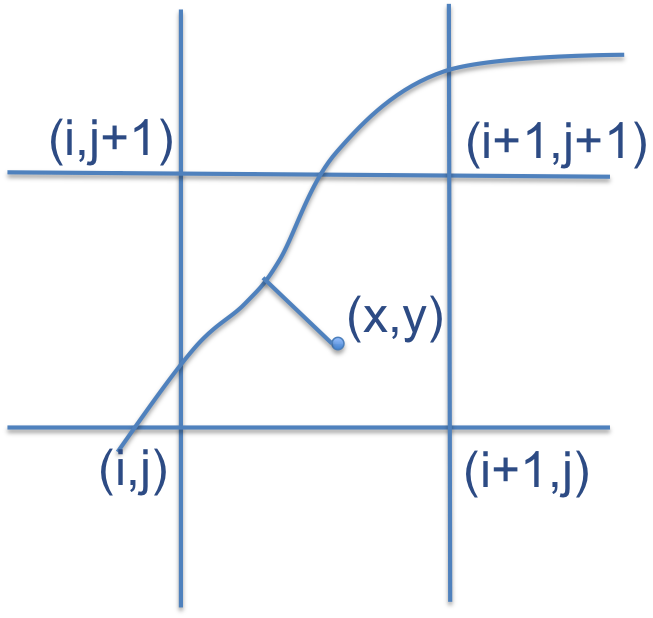## Create a level set in OpenVDB

So lets create a level set of a cylinder with center in the origin and z-axis oriented. In order to do that, we need to specify the radius of the cylinder, the grid dimensions, and the voxel (cell of the grid) size. Pay attention that in OpenVDB you have an index space for your grid and a world space. Index space for the grid is , note that index might be negative. If (i, j, k) is a point in the index space, then is the point in the world space, where is a vector of voxel sizes. The code below creates a grid which contains floats, specifies bounding box of our level set in the index space, and pass everything to makeCylinder. For simplicity uniform grid is used so . In order to modify elements of the grid, accessor object is used. Inside, we have loop on all points in the grid where we compute value of the function. In order to use our grid further, we need to define interpolator which will be used with this grid. It is done by calling method setTransform. It is easy to see that makeCylinder might be generalized by introducing additional argument of type std::function\<float (const Vec3d&)\> lsFunc (in C++11) which allows to fill in a grid by any signed distance level set function.

After running this application, you should get something like that: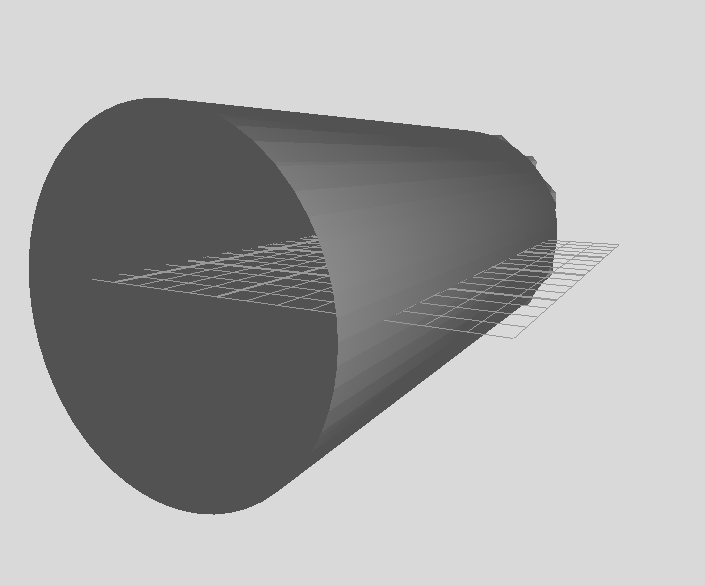## Create a narrow-band level set

It is cool to be able to create and save a regular grid in the file. Yet there is one pitfall - the file containing simple cylinder is 2.67MB in size:

As you might guess there are a lot information in the grid which we are not interested in - all grid vertices which are far away from the surface . OpenVDB allows to store only points which are in vicinity of the surface. The code below demonstrates it.

For every point it saves a value in the grid only if . In the code, backgroundValue specifies the module of this distance. Note, that the call of the method signedFloodFill() propagates the sign from initialized grid points to the uninitialized, since the background value is set in the grid by module. signedFloodFill() might be used on closed surfaces only, so I picked up a sphere instead of a cylinder. If you run this code and use vdb_print to check out the information about grid you will get that the grid is 27x27x27 instead of 40x40x40. For a more optimal code for sphere generation, check out openvdb/tools/LevelSetSphere.h.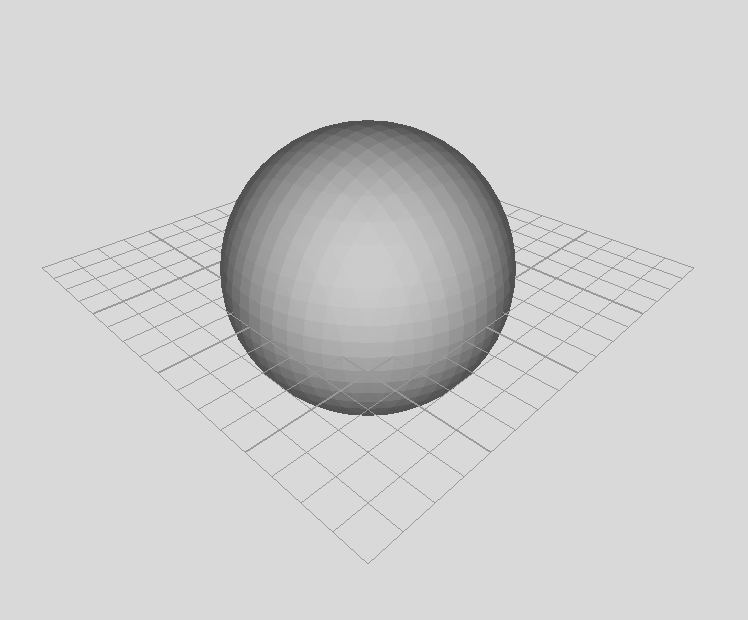## Read the grid and interpolate a level set function value in a point

Reading the grid is straight forward, the code below demonstrates it. Pay attention how to use linear interpolator in order to find a value of the level set function for an arbitrary point. Venusstatue.vdb is available here.

In the next part I will write about more advanced things such as deforming level set surfaces using PDE.

If you have any questions / suggestions about this material or a collaboration idea regarding level sets / openvdb, feel free to contact me.